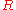xJoule's lawEncyclopedia
Joule's laws are a pair of laws concerning the heat produced by a current and the energy dependence of an ideal gas
Ideal gas
An ideal gas is a theoretical gas composed of a set of randomly-moving, non-interacting point particles. The ideal gas concept is useful because it obeys the ideal gas law, a simplified equation of state, and is amenable to analysis under statistical mechanics.At normal conditions such as...

to that of pressure, volume, and temperature, respectively. They are named after James Prescott Joule
James Prescott Joule
James Prescott Joule FRS was an English physicist and brewer, born in Salford, Lancashire. Joule studied the nature of heat, and discovered its relationship to mechanical work . This led to the theory of conservation of energy, which led to the development of the first law of thermodynamics. The...

.

Joule's first law, also known as the Joule effect, is a physical law
Physical law
A physical law or scientific law is "a theoretical principle deduced from particular facts, applicable to a defined group or class of phenomena, and expressible by the statement that a particular phenomenon always occurs if certain conditions be present." Physical laws are typically conclusions...

expressing the relationship between the heat
Heat
In physics and thermodynamics, heat is energy transferred from one body, region, or thermodynamic system to another due to thermal contact or thermal radiation when the systems are at different temperatures. It is often described as one of the fundamental processes of energy transfer between...

generated by the current
Electric current
Electric current is a flow of electric charge through a medium.This charge is typically carried by moving electrons in a conductor such as wire...

flowing through a conductor. Joule studied this phenomenon in the 1840s. It is expressed as:where Q is the heat generated by a constant current
Electric current
Electric current is a flow of electric charge through a medium.This charge is typically carried by moving electrons in a conductor such as wire...

I flowing through a conductor of electrical resistance
Electrical resistance
The electrical resistance of an electrical element is the opposition to the passage of an electric current through that element; the inverse quantity is electrical conductance, the ease at which an electric current passes. Electrical resistance shares some conceptual parallels with the mechanical...

R, for a time
Time
Time is a part of the measuring system used to sequence events, to compare the durations of events and the intervals between them, and to quantify rates of change such as the motions of objects....

t. When current, resistance and time are expressed in ampere
Ampere
The ampere , often shortened to amp, is the SI unit of electric current and is one of the seven SI base units. It is named after André-Marie Ampère , French mathematician and physicist, considered the father of electrodynamics...

s, ohms, and second
Second
The second is a unit of measurement of time, and is the International System of Units base unit of time. It may be measured using a clock....

s respectively, the unit
Units of energy
Because energy is defined via work, the SI unit for energy is the same as the unit of work – the joule , named in honour of James Prescott Joule and his experiments on the mechanical equivalent of heat...

of Q is the joule
Joule
The joule ; symbol J) is a derived unit of energy or work in the International System of Units. It is equal to the energy expended in applying a force of one newton through a distance of one metre , or in passing an electric current of one ampere through a resistance of one ohm for one second...

. Joule's first law is sometimes called the Joule–Lenz law since it was later independently discovered by Heinrich Lenz
Heinrich Lenz
Heinrich Friedrich Emil Lenz was a Russian physicist of Baltic German ethnicity. He is most noted for formulating Lenz's law in electrodynamics in 1833....

. The heating effect of conductors carrying currents is known as Joule heating
Joule heating
Joule heating, also known as ohmic heating and resistive heating, is the process by which the passage of an electric current through a conductor releases heat. It was first studied by James Prescott Joule in 1841. Joule immersed a length of wire in a fixed mass of water and measured the temperature...

.

Joule's second law states that the internal energy
Internal energy
In thermodynamics, the internal energy is the total energy contained by a thermodynamic system. It is the energy needed to create the system, but excludes the energy to displace the system's surroundings, any energy associated with a move as a whole, or due to external force fields. Internal...

of an ideal gas
Ideal gas
An ideal gas is a theoretical gas composed of a set of randomly-moving, non-interacting point particles. The ideal gas concept is useful because it obeys the ideal gas law, a simplified equation of state, and is amenable to analysis under statistical mechanics.At normal conditions such as...

is independent of its volume and pressure, depending only on its temperature.

## Relation to Ohm's law

In the context of resistive circuits and in light of conservation of energy
Conservation of energy
The nineteenth century law of conservation of energy is a law of physics. It states that the total amount of energy in an isolated system remains constant over time. The total energy is said to be conserved over time...

and electrical potential, Joule's first law and Ohm's law
Ohm's law
Ohm's law states that the current through a conductor between two points is directly proportional to the potential difference across the two points...

are equivalent and derivable from each other (as explained by James Clerk Maxwell
James Clerk Maxwell
James Clerk Maxwell of Glenlair was a Scottish physicist and mathematician. His most prominent achievement was formulating classical electromagnetic theory. This united all previously unrelated observations, experiments and equations of electricity, magnetism and optics into a consistent theory...

in 1881,
by Mascart
Éleuthère Mascart
Éleuthère Élie Nicolas Mascart was a noted French physicist, a researcher in optics and electricity.Mascart was born in Quarouble, Nord. He attended the École normale supérieure...

in 1883, and by Oliver Heaviside
Oliver Heaviside
Oliver Heaviside was a self-taught English electrical engineer, mathematician, and physicist who adapted complex numbers to the study of electrical circuits, invented mathematical techniques to the solution of differential equations , reformulated Maxwell's field equations in terms of electric and...

in 1894), though they were discovered independently and experimentally, before the notions of conservation of energy and electrical potential were well developed.

Joule's first law states that the rate of heat
Heat
In physics and thermodynamics, heat is energy transferred from one body, region, or thermodynamic system to another due to thermal contact or thermal radiation when the systems are at different temperatures. It is often described as one of the fundamental processes of energy transfer between...

dissipation
Dissipation
In physics, dissipation embodies the concept of a dynamical system where important mechanical models, such as waves or oscillations, lose energy over time, typically from friction or turbulence. The lost energy converts into heat, which raises the temperature of the system. Such systems are called...

in a resistive conductor
Electrical conductor
In physics and electrical engineering, a conductor is a material which contains movable electric charges. In metallic conductors such as copper or aluminum, the movable charged particles are electrons...

is proportional to the square of the current
Electric current
Electric current is a flow of electric charge through a medium.This charge is typically carried by moving electrons in a conductor such as wire...

through it and to its resistance
Electrical resistance
The electrical resistance of an electrical element is the opposition to the passage of an electric current through that element; the inverse quantity is electrical conductance, the ease at which an electric current passes. Electrical resistance shares some conceptual parallels with the mechanical...

. That is, the power dissipated in a resistor, in terms of the current through it and its resistance, is: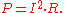Joule arrived at this result experimentally in 1841, using a calorimeter
Calorimeter
A calorimeter is a device used for calorimetry, the science of measuring the heat of chemical reactions or physical changes as well as heat capacity. Differential scanning calorimeters, isothermal microcalorimeters, titration calorimeters and accelerated rate calorimeters are among the most common...

to measure heat, and a galvanometer
Galvanometer
A galvanometer is a type of ammeter: an instrument for detecting and measuring electric current. It is an analog electromechanical transducer that produces a rotary deflection of some type of pointer in response to electric current flowing through its coil in a magnetic field. .Galvanometers were...

to measure current, with a variety of resistive circuits.

The law applies to any circuit that obeys Ohm's law
Ohm's law
Ohm's law states that the current through a conductor between two points is directly proportional to the potential difference across the two points...

, that is, that conducts a current proportional to the voltage
Voltage
Voltage, otherwise known as electrical potential difference or electric tension is the difference in electric potential between two points — or the difference in electric potential energy per unit charge between two points...

across it, or equivalently, that can be characterized by a resistance. Ohm's law states that for a voltage V across a circuit of resistance R the current will be: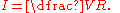By substituting this formula for current into one or both factors of current in Joule's law, the power dissipated can be written in the equivalent forms: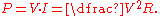The relation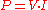is actually more generally applicable than either Joule's law or Ohm's law, as it represents the instantaneous power
Power (physics)
In physics, power is the rate at which energy is transferred, used, or transformed. For example, the rate at which a light bulb transforms electrical energy into heat and light is measured in watts—the more wattage, the more power, or equivalently the more electrical energy is used per unit...

being applied to a circuit with voltage V across it and current I into it, whether the circuit is resistive or not. In combination with either Ohm's law or Joule's law, it may be used to derive the other.

Since the power
Power (physics)
In physics, power is the rate at which energy is transferred, used, or transformed. For example, the rate at which a light bulb transforms electrical energy into heat and light is measured in watts—the more wattage, the more power, or equivalently the more electrical energy is used per unit...

dissipated by a resistor is the amount of energy
Energy
In physics, energy is an indirectly observed quantity. It is often understood as the ability a physical system has to do work on other physical systems...

used (electrical work
Work (thermodynamics)
In thermodynamics, work performed by a system is the energy transferred to another system that is measured by the external generalized mechanical constraints on the system. As such, thermodynamic work is a generalization of the concept of mechanical work in mechanics. Thermodynamic work encompasses...

applied) per unit time, the total energy consumed and dissipated in time t is: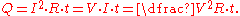## Hydraulic equivalent

In the energy balance of groundwater flow
Groundwater energy balance
The groundwater energy balance is the energy balance of a groundwater body in terms of incoming hydraulic energy associated with groundwater inflow into the body, energy associated with the outflow, energy conversion into heat due to friction of flow, and the resulting change of energy status and...

Darcy's law
Darcy's law is a phenomenologically derived constitutive equation that describes the flow of a fluid through a porous medium. The law was formulated by Henry Darcy based on the results of experiments on the flow of water through beds of sand...

) an hydraulic equivalent of Joule's law is used :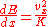where: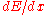= loss of hydraulic energy (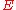) due to friction of flow in-direction per unit of time (m/day) – comparable to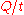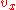= flow velocity in-direction (m/day) – comparable to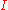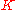= hydraulic conductivity
Hydraulic conductivity
Hydraulic conductivity, symbolically represented as K, is a property of vascular plants, soil or rock, that describes the ease with which water can move through pore spaces or fractures. It depends on the intrinsic permeability of the material and on the degree of saturation...

of the soil (m/day) – the hydraulic conductivity is inversely proportional to the hydraulic resistance which compares to